###Dimensional Analysis

The Gravitational Constant

Newton's law of gravity is usuallyexpressed in terms of a universal constant, G: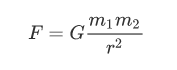The universal constants c,h,G can be usedto re-express the fundamental units for mass, time and distance. That meansthat any physical quantity in mechanics can be expressed in terms of thesethree constants. In the case of thermodynamics or electrodynamics, there are afew other universal constants that would need to be included to have a completedescription.

Recall that: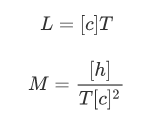If we replace this in the relation weobtained in the above exercise, we get: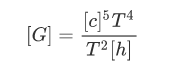So we can get a relation for the time dimension:or: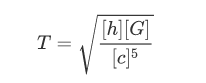Replacing the value for T in terms of[h],[c],[G] in the above equations we get: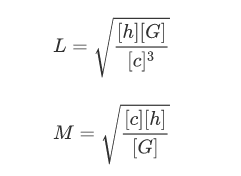Based on these dimensional relations we canintroduce three new units for distance, time and mass, defined exclusively interms of these three universal constants: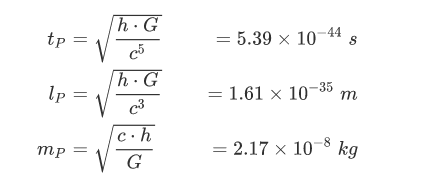Because these units are defined in terms ofuniversal physical constants, we expect them to have a deeper physical meaningcompared to our standard units - the second, the meter and the kilogram:

the second has been defined as the 1/(243600) part of a mean solar day;

the meter has been defined as the 1/40106 part of the circumference of the Earth through thepoles;

the kilogram has been defined as the 1/1000part of a volume of one cubic meter of pure water at the temperature of meltingice.

While the precise definitions of thesecond, meter and kilogram have slightly changed, their values areapproximately the same. While they were designed to unify and standardizeprevious units, their definition is far from universal. These units impose ascale fitted for the human perception of space, time and weight.

The base Planck units impose a scale with amore profound physical meaning and implications.